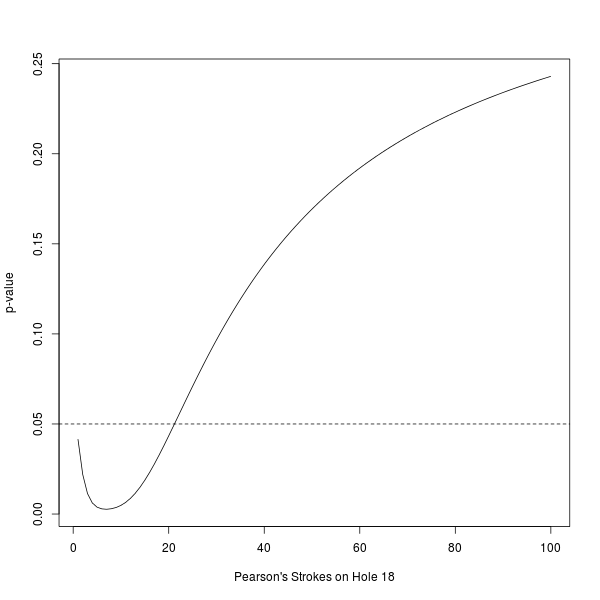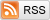# T-Tests Aren't Monotonic

October 22, 2014

R. A. Fisher and Karl Pearson play a heated round of golf. Being Statisticians, they agree before the round to run a two-sided paired T-test to see if either of them is statistically significantly better. After the first 17 holes, Fisher is ahead by 19 strokes, and openly gloating. On the 18th hole, he sinks a 20-foot putt for birdey, and smirks at Pearson. Pearson then "accidentally" hits his ball into several sand traps, trees, and water hazards, taking 100 strokes on the last hole.

When he finally sinks the ball, after a series of disastrous putts, Pearson turns and says, "Wow, that last hole was tricky, eh Ronald? And would you look at that! Seems the p-value for the two-sided paired T-test is only 0.24, not statistically significant... well, better luck next time!"

This scenario could actually have happened, or at least, the numerical part of it could:

```> fisher  <- c(3, 3, 4, 5, 4, 3, 4, 5, 4, 6, 4, 5, 5, 5, 4, 5, 5, 4)
> pearson <- c(4, 4, 3, 7, 6, 4, 5, 6, 8, 5, 6, 8, 4, 6, 7, 5, 5, 100)
> sum(pearson[1:17]) - sum(fisher[1:17])
 19
> t.test(fisher, pearson, paired=TRUE)\$p.value
 0.242975
```

This surprisingly large p-value stems from the T-statistic not being monotonically increasing as a function of its inputs. Indeed, had Pearson played more competitively on his last hole, the T-test would have identified Fisher as the clearly superior golfer:

```> pearson <- 5
> t.test(fisher, pearson, paired=TRUE)\$p.value
 0.003844234
```

The numerical reason for this is that the outlier increases not only the sample mean, but also the standard error. The sample mean is an estimate of the effect size, and the standard error represents the uncertainty associated with the estimated effect size. The ratio of these quantities is the T-statistic, which we then use to get a p-value. As the outlier gets larger, the uncertainty effect outweighs the sample mean effect.

To get a more complete picture, we can plot the p-value of the T-test as a function of Pearson's strokes on the last hole. (The dashed line represents the holy, not-at-all-arbitrary 0.05 significance level).

```> p.value <- function(x){t.test(fisher, c(pearson[1:17], x), paired=TRUE)\$p.value}
> plot(1:100, sapply(1:100, p.value), xlab="Pearson's Strokes on Hole 18", ylab="p-value", type="l")
> abline(h=0.05, lty="dashed")
```As you can see, the p-value of the T-test is not simply a decreasing function of Pearson's strokes on the last hole. (In our scenario, Pearson is losing by so much after 17 holes that the non-monotonicity induced by using a two-sided test has no effect in the range of values we plotted).

Interestingly, the p-value is minimized when Pearson has 7 strokes. In other words, the non-monotonicity "kicks in" quite early! Indeed, Pearson could have prevented statistical significance with only 22 strokes on his last hole, substantially lessening the strain on his acting skills.

With a little mental math, you can figure out the limiting p-value as you take Pearson's strokes on the last hole to infinity. In fact, the T-statistic goes to exactly 1, (regardless of n, the number of sample points), and so the limiting two-sided p-value is exactly equal to the probability that a T-distributed random variable with n-1 degrees of freedom would exceed 1 in absolute value. In our case with n=18, this limit is

```> 2 * pt(q=1, df=18-1, lower.tail=FALSE)
 0.3313328
```

How should we interpret this unusual behavior in such a popular test? One interpretation is that the T-test is designed to operate on normally distributed random variables. Sometimes, it also works reasonably on not-too-skewed random variables, through the central limit theorem. But it isn't designed to work for random variables with a distribution as skewed as the difference in strokes between Pearson and Fisher. And it certainly isn't designed to work in the presence of adversarial action like that which Pearson employed.

To be fair, if you truly believed that the stroke differences between Pearson and Fisher were normally distributed, (though they're clearly not, most obviously because they are all small integers), then the T-test would in fact be a reasonable test to use. And, if you actually had this much faith, then the non-monotonicity might be a reasonable property.

For this adversarial situation, though, a better test might be the sign test. Under this test, we assume that under the null hypothesis Pearson and Fisher are equally likely to win a hole. Then, the number of times that Fisher beat Pearson, conditional on the number of times Fisher and Pearson didn't tie, is binomially distributed with success probability 1/2. Under this test, we get a statistically significant two-sided p-value of 0.02:

```> 2 * pbinom(q=sum(fisher > pearson), size=sum(pearson != fisher), prob=0.5)
 0.02127075
```

Under the sign test, the one-sided p-value is a non-increasing function of each of its inputs. In our case, this means that it incentivizes Pearson and Fisher to both play competitively. Unfortunately, under the normal distribution the sign test is not as powerful as the T-test; asymptotically it requires 1.57 (pi/2) times as much data as the T-test to achieve the same power.